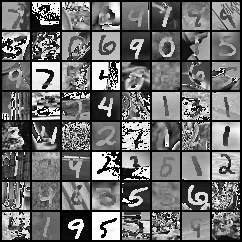# Convert RGB to gray?

`torchvision.transforms.functional.to_grayscale()` can only applied to PIL Image.
then how can I convert torch.Tensor RGB to gray?

You can convert the Tensor to a PIL image, apply that transform, then convert it back to a Tensor.

2 Likes

Well, like @etekiller says, you can try to “Compose Transforms”. An example would be here. And then use image to PIL transform to solve your issue. This though will likely add a ton of time overhead to your data loading pipeline!

If you’re not using many transforms from pytorch on your data during “Composing your transform”, why not try using normal tensor operations to go from RGB to Gray? Try the function OpenCV uses like here. You can find the reasons for such an averaging here

3 Likes

@shubhvachher @etekiller
Thanks a lot. actually, I want to convert MNIST-M channel 3 to 1.
Easiest way to make this is using transforms.Compose() like this.

``````    pre_process = transforms.Compose(
[transforms.Grayscale(num_output_channels=1), transforms.ToTensor(),
transforms.Normalize(mean=[0.5], std=[0.5])])
``````But I have to do this in dataloader for loop statement, so I write like this.
and there are some differences between two methods.

``````    pre_process = transforms.Compose(
[transforms.ToTensor(),
transforms.Normalize(mean=[0.5, 0.5, 0.5], std=[0.5, 0.5, 0.5])])

for i, (images, labels) in enumerate(loader_mnistm):
images, labels = images.to(device), labels.to(device)

images = images.cpu()
images = [torchvision.transforms.ToPILImage()(x) for x in images]
images = [torchvision.transforms.Grayscale()(x) for x in images]
images = [torchvision.transforms.ToTensor()(x) for x in images]
images = torch.stack(images).to(device)
``````What`s wrong with my code?

Sorry, I am not sure I fully get what you’re trying to do. If you simply want to apply those transforms to all images you can just pass the transforms into the Dataset’s (or any of the child classes) constructor parameter.

In my case I pass it like this:

``````dataset_in = datasets.ImageFolder('./rooms', transform = transform_in )
``````

Where `transform_in` is the transform created via Compose.

Finally I Solve it.
Thanks a lot !

Hi, what was the issue and how did you solve it?